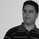‎12792‎ مشاهدة
12792
This is my second Version of Heikin Ashi Strategy.

Changes:
-Different Time Frame for Fast EMA and Slow EMA
-MACD Filter with own Time Frame

You can tweak around with settings , but defaults gives good results in Forex,Stocks and Index.

```//@version=2
//Heikin Ashi Strategy  V2 by breizh29

strategy("Heikin Ashi Strategy  V2",shorttitle="HAS V2",overlay=true,default_qty_value=1000,initial_capital=100000,currency=currency.EUR)
res = input(title="Heikin Ashi Candle Time Frame", type=resolution, defval="60")
hshift = input(1,title="Heikin Ashi Candle Time Frame Shift")
res1 = input(title="Heikin Ashi EMA Time Frame", type=resolution, defval="180")
mhshift = input(0,title="Heikin Ashi EMA Time Frame Shift")
fama = input(1,"Heikin Ashi EMA Period")
test = input(1,"Heikin Ashi EMA Shift")
sloma = input(30,"Slow EMA Period")
slomas = input(1,"Slow EMA Shift")
macdf = input(false,title="With MACD filter")
res2 = input(title="MACD Time Frame", type=resolution, defval="15")
macds = input(1,title="MACD Shift")

//Heikin Ashi Open/Close Price
ha_t = heikinashi(tickerid)
ha_open = security(ha_t, res, open[hshift])
ha_close = security(ha_t, res, close[hshift])
mha_close = security(ha_t, res1, close[mhshift])

//macd
[macdLine, signalLine, histLine] = macd(close, 12, 26, 9)
macdl = security(ha_t,res2,macdLine[macds])
macdsl= security(ha_t,res2,signalLine[macds])

//Moving Average
fma = ema(mha_close[test],fama)
sma = ema(ha_close[slomas],sloma)
plot(fma,title="MA",color=lime,linewidth=2,style=line)
plot(sma,title="SMA",color=red,linewidth=2,style=line)

//Strategy
golong =  crossover(fma,sma) and (macdl > macdsl or macdf == false )
goshort =   crossunder(fma,sma) and (macdl < macdsl or macdf == false )

strategy.entry("Sell",strategy.short,when = goshort)

```Hello,
may you please precise how the "ongoing" fma is calculated?
Let's assume the n-1 period of 180 minutes is ended. We are entering in the new period of 180, which consists of 12 periods of 15 minutes. Plotting the fma on 15 minutes timeframe, I do see it is calculated "ongoing", but I do not understand what "close" and "previus EMA" values it is considering (EMA formula = * (2 / n+1) + previous EMA.)
Thanks,
Aldo
ردThere is a bug in this strategy. This strategy is trading by looking at the future closing price.
Example : 24 Mar 16' 22:15, fma is 1.1169. But, fma has a higher value when calculated from open, high, low, close in the past 3 hours.
(this calculation of this strategy includes 25 Mar 16' 00:45)

But you can still get profits.
ردHi, very good trading system. Have you this trading system to MT5? Thank's.
رد